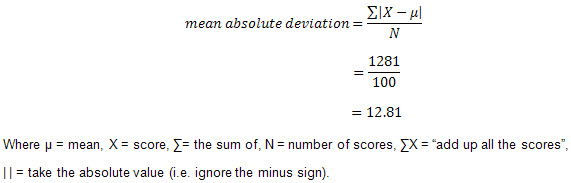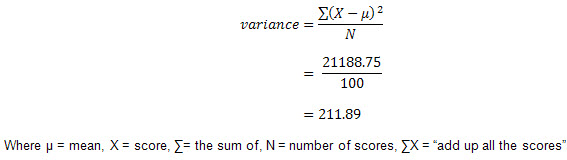# Absolute Deviation & Variance

## Variation

Quartiles are useful, but they are also somewhat limited because they do not take into account every score in our group of data. To get a more representative idea of spread we need to take into account the actual values of each score in a data set. The absolute deviation, variance and standard deviation are such measures.

The absolute and mean absolute deviation show the amount of deviation (variation) that occurs around the mean score. To find the total variability in our group of data, we simply add up the deviation of each score from the mean. The average deviation of a score can then be calculated by dividing this total by the number of scores. How we calculate the deviation of a score from the mean depends on our choice of statistic, whether we use absolute deviation, variance or standard deviation.

## Absolute Deviation and Mean Absolute Deviation

Perhaps the simplest way of calculating the deviation of a score from the mean is to take each score and minus the mean score. For example, the mean score for the group of 100 students we used earlier was 58.75 out of 100. Therefore, if we took a student that scored 60 out of 100, the deviation of a score from the mean is 60 - 58.75 = 1.25. It is important to note that scores above the mean have positive deviations (as demonstrated above), whilst scores below the mean will have negative deviations.

To find out the total variability in our data set, we would perform this calculation for all of the 100 students' scores. However, the problem is that because we have both positive and minus signs, when we add up all of these deviations, they cancel each other out, giving us a total deviation of zero. Since we are only interested in the deviations of the scores and not whether they are above or below the mean score, we can ignore the minus sign and take only the absolute value, giving us the absolute deviation. Adding up all of these absolute deviations and dividing them by the total number of scores then gives us the mean absolute deviation (see below). Therefore, for our 100 students the mean absolute deviation is 12.81, as shown below:Join the 10,000s of students, academics and professionals who rely on Laerd Statistics.

## Variance

Another method for calculating the deviation of a group of scores from the mean, such as the 100 students we used earlier, is to use the variance. Unlike the absolute deviation, which uses the absolute value of the deviation in order to "rid itself" of the negative values, the variance achieves positive values by squaring each of the deviations instead. Adding up these squared deviations gives us the sum of squares, which we can then divide by the total number of scores in our group of data (in other words, 100 because there are 100 students) to find the variance (see below). Therefore, for our 100 students, the variance is 211.89, as shown below:As a measure of variability, the variance is useful. If the scores in our group of data are spread out, the variance will be a large number. Conversely, if the scores are spread closely around the mean, the variance will be a smaller number. However, there are two potential problems with the variance. First, because the deviations of scores from the mean are 'squared', this gives more weight to extreme scores. If our data contains outliers (in other words, one or a small number of scores that are particularly far away from the mean and perhaps do not represent well our data as a whole), this can give undo weight to these scores. Secondly, the variance is not in the same units as the scores in our data set: variance is measured in the units squared. This means we cannot place it on our frequency distribution and cannot directly relate its value to the values in our data set. Therefore, the figure of 211.89, our variance, appears somewhat arbitrary. Calculating the standard deviation rather than the variance rectifies this problem. Nonetheless, analysing variance is extremely important in some statistical analyses, discussed in other statistical guides.